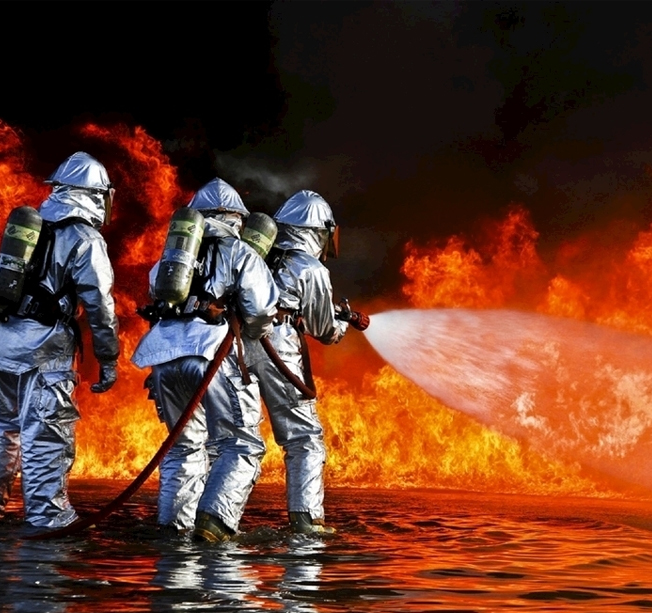36000 + 展会面积 450 + 展商 300 + 媒体争相报道 20000 + 专业观众

# var now = new Date(); function GetServerTime() { var urodz = new Date("7/28/2021 00:00:00");//设定倒计时的时间 now.setTime(now.getTime()+250); days = (urodz - now) / 1000 / 60 / 60 / 24; daysRound = Math.floor(days); hours = (urodz - now) / 1000 / 60 / 60 - (24 * daysRound); hoursRound = Math.floor(hours); minutes = (urodz - now) / 1000 /60 - (24 * 60 * daysRound) - (60 * hoursRound); minutesRound = Math.floor(minutes); seconds = (urodz - now) / 1000 - (24 * 60 * 60 * daysRound) - (60 * 60 * hoursRound) - (60 * minutesRound); secondsRound = Math.round(seconds); var obj = document.getElementById("time_second"); daysRound = daysRound; if(daysRound >= 0) { obj.innerHTML=""+daysRound+"天" ; }else { obj.innerHTML="0天" ; } } setInterval("GetServerTime()",1000); //\$(function() { GetServerTime() //});